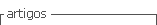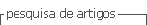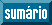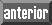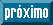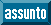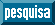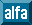## Artigo

•Citado por SciELO

## versão impressa ISSN 0379-9123versão On-line ISSN 2617-4731

#### Resumo

DIAZ LEZCANO, Maura Isabel  e  HEYN CHAPARRO, Jaime Montgomery. Estimation of carbon content in the forest coverage of a secondary forest of the district of Curuguaty, Paraguay. Rev. Soc. cient. Parag. [online]. 2022, vol.27, n.2, pp.55-71. ISSN 2617-4731.  https://doi.org/10.32480/rscp.2022.27.2.55.

Abstract: Paraguay is located in position 162 among the countries that emit greenhouse gases (GHG), which, as a member of the group of developing countries, needs to ensure its national climate change strategy, with adaptation programs incorporated into mitigation programs within the development process that make it possible to maintain or reduce our emissions. The objective of this research was to evaluate the carbon storage of a secondary forest in the Curuguaty District, Canindeyú Department, Paraguay. The methodology consisted in the installation of 4 plots of 50 x 50 m (10,000 m2) totaling a study area of 1 ha, individuals with DBH ≥ 10 cm were inventoried, data on the floristic composition were taken, and the results were analyzed at Through descriptive statistics to determine the basal area and stem volume, the aerial, root and total carbon (C) and the equivalent carbon were estimated later, using the allometric equations 1 and 2. The results obtained were: 21 families, 17 genera, 22 species and 249 individuals / ha. The basal area gave an average of 17.1 m2 / ha, the average stem volume was 16.5 m3 / ha. For the determination of biomass, the estimated values were 59.2 tC / ha (equation 1) and 37.3 t / ha (equation 2). The total biomass according to the equation 1 was 118.5 t / ha and according to the estimates of the allometric equations of equation 2 of 74 t / ha. For total carbon, they were carried out using the equation 1, the average carbon stored was 59.2 tC / ha with a confidence level of 95%. On the other hand, the results obtained from the allometric equation of equation 2, was 37.3 tC / ha. The existence of significant differences between the two equations applied was verified. The carbon dioxide estimated according to the equation 1 equation was 219 tCO2e / ha and equation 2 136.7 tCO2e / ha. It is concluded that the secondary forest located in the urban area of the Curuguaty District constitutes an important carbon sink that contributes to the mitigation of greenhouse gases.

Palavras-chave : biomass; allometric equations; floristic composition; greenhouse gases..

· resumo em Espanhol     · texto em Espanhol     · Espanhol (pdf )